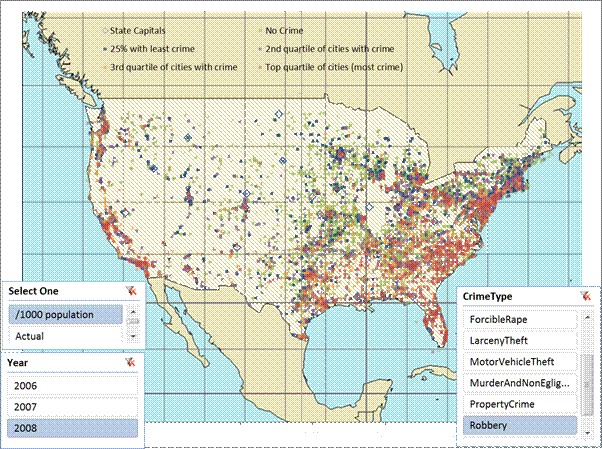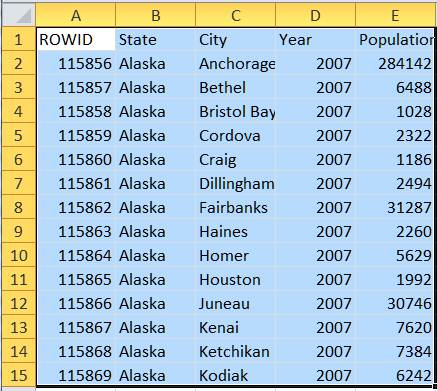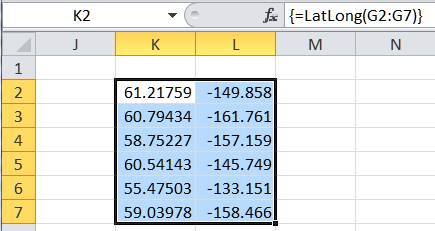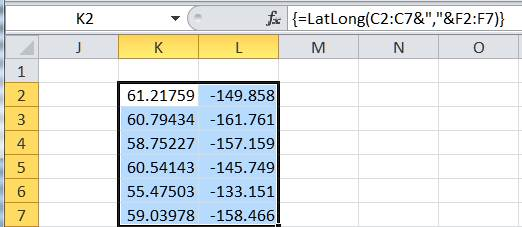Home > Publications & Training > Data Visualization > 10a Dashboards-GeocodingShare Your

## Geocoding and Maps

Before one can position an address (a street address or a town itself) on a map, it must be converted to geographic coordinates (latitude and longitude).  Then, that latitude and longitude is mapped to a particular location on the map.  Figure 1 shows several thousand U.S. cities and towns on a Mercator projection map.  Well, it’s actually an Excel XY Scatter chart made to look like a map of the U.S.  It plots the crime rate for a specific crime for a specific year but that’s not the focus of this note.  Here we focus on geocoding an address using Excel and VBA and plotting the resulting geographic coordinates (latitude and longitude) onto a map.Figure 1 – This is a XY Scatter chart!

Before locating a town on the map, one must know its geographic coordinates, i.e., the latitude and longitude.  The process of converting an address to coordinates is known as geocoding.

### A Geocoding API

The code below requires references to the Microsoft XML library and the Microsoft VBScript Regular Expressions library.

A quick search of the web led me to a Google API, which looked like it was easy enough to use.  The call was easy enough.  Calling http://maps.googleapis.com/maps/api/geocode/json?address=Mountain+View,CA&sensor=false returns a variety of information about the address in the JSON format.  After developing the code  to use the API and parse the response, I discovered that Google limits the number of calls to the service to 2,500 a day.  That meant there was no way I could map all the 8,000 or so towns for which I had data.

Luckily, I discovered Yahoo! had no such limit – or, the limit was sufficiently large that I did not reach it.  The Yahoo! API was also easy enough: http://api.maps.yahoo.com/ajax/geocode?appid=onestep&qt=1&id=m&qs=mountain+view,ca  The JSON response was short: YGeoCode.getMap({"GeoID":"m","GeoAddress":"mountain view, ca","GeoPoint":{"Lat":37.389475,"Lon":-122.081694},"GeoMID":false,"success":1},1); .  Consequently, I decided to simply parse the returned string rather than convert the JSON text to an object and query the appropriate object property.  So, the call to the web service looked like:

Private Function processOneCity(sInput As String)

Static oHttp As XMLHTTP

If oHttp Is Nothing Then Set oHttp = New XMLHTTP

oHttp.Open "GET", _

"http://api.maps.yahoo.com/ajax/geocode?appid=onestep&qt=1&id=m&qs=" _

& URLEncode(sInput, True), False

oHttp.send

Code Sample 1

Declaring the oHTTP object as static meant it did not have to be initialized on each call.  The URLEncode function converts every token that cannot be included in a URL into its web-safe hex equivalent for inclusion in the URL.  The 2nd parameter being False informs the system that the call will be synchronous and that our code will wait for the result from Yahoo!

If the web service returns a valid response, the code parses out the latitude and longitude into a 2 element array named Rslt.  After locating the character position after the “GeoPoint” literal, the task of individually extracting the latitude and longitude is delegated to the parseOneToken function.

If oHttp.Status = 200 Then

Dim ResponseText As String: ResponseText = oHttp.ResponseText

Dim Idx As Long

Idx = InStr(1, ResponseText, """GeoPoint""", vbTextCompare) _

+ Len("""GeoPoint""")

Dim Rslt(1) As Single

Rslt(0) = parseOneToken(Mid(ResponseText, Idx), """lat""")

Rslt(1) = parseOneToken(Mid(ResponseText, Idx), """Lon""")

processOneCity = Rslt

End If

End Function

Code Sample 2

The 2 support functions, URLEncode and parseOneToken are in Code Sample 3.  URLEncode, as already mentioned, makes a string safe for use in a URL.  The parseOneToken function uses a regular expression object to extract all the numbers after the token specified in the aToken argument.

Public Function URLEncode(StringToEncode As String, _

Optional UsePlusForSpace As Boolean = True) As String

Dim TempAns As String

Dim I As Long

For I = 1 To Len(StringToEncode)

Dim aChar As String: aChar = Mid(StringToEncode, I, 1)

Select Case aChar

Case "a" To "z", "A" To "Z", "0" To "9":

TempAns = TempAns & aChar

Case " ":

TempAns = TempAns & IIf(UsePlusForSpace, "+", "%" & Hex(Asc(" ")))

Case Else:

TempAns = TempAns & "%" & _

Right("0" & Hex(Asc(aChar)), 2)

End Select

Next I

URLEncode = TempAns

End Function

Private Function parseOneToken(ByVal Str As String, ByVal aToken As String)

Dim Idx As Long: Idx = InStr(1, Str, aToken, vbTextCompare)

Idx = Idx + Len(aToken)

Idx = InStr(Idx, Str, ":") + 1

Do While Mid(Str, Idx, 1) = " ": Idx = Idx + 1: Loop

Static RE As RegExp: If RE Is Nothing Then Set RE = New RegExp

RE.Global = False

RE.Pattern = "-*[0-9]*(\.[0-9]*)*"

Dim Rslt As MatchCollection

Set Rslt = RE.Execute(Mid(Str, Idx))

parseOneToken = Rslt(0)

End Function

### Defining the User Defined Function

With that research out of the way, I turned to the data set I was using.  It contained a list of cities and their characteristics such as the population.  A snapshot of the dataset is in Figure 2.Since I also had a list of state names and their corresponding state abbreviations, I used a VLOOKUP function to add a column with the state abbreviation and, for convenience, create a “full address” in another column.Figure 3

At this stage I envisioned two different ways to call the UDF, each with two variants.  To start with, I named the new function LatLong.

First, one could call it with a single address such as a cell reference that contains {city},{state abbreviation} as in =LatLong(G2) or where the address is the result of a formula as in =LatLong(C2&”,”&F2).

The second way would be to pass multiple addresses and in return the function would return multiple coordinates, one for each address.  This requires the function to be “array aware.”  Here, as in the case of a single address, there are two variants.  The first would be to use the function with cells that already contain the complete address; the other would be to create the addresses with a formula.### Creating the UDF

Catering to the different ways to call the UDF LatLong requires processing three different types of inputs: a simple string (the input is a single address), a 1D array (multiple addresses each of which is in an individual cell as in Figure 4), and a 2D array (multiple addresses each of which is the result of a formula as in Figure 5).  The LatLong function handles the aspects of the array formulas delegating the task of actually processing a single address to another function, doOneCity.  The MapInput and MapOutput functions are responsible for ensuring that the input and output data are structured correctly.

Public Function LatLong(ByVal sInput)

Dim Arr: Arr = MapInput(sInput)

Dim Rslt(), I As Long

Select Case NbrDim(Arr)

Case 2:

ReDim Rslt(ArrLen(Arr) - 1)

For I = LBound(Arr) To UBound(Arr)

Rslt(I - LBound(Arr)) = doOneCity(CStr(Arr(I, 1)))

Next I

LatLong = MapOutput(Rslt)

Case 1:

ReDim Rslt(ArrLen(Arr) - 1)

For I = LBound(Arr) To UBound(Arr)

Rslt(I - LBound(Arr)) = doOneCity(CStr(Arr(I)))

Next I

LatLong = MapOutput(Rslt)

Case Else:

LatLong = MapOutput(doOneCity(CStr(Arr)))

End Select

End Function

Code Sample 4

I decided to incorporate a caching mechanism in the doOneCity function.  With the cache active, the function calls the Web service only the first time that it encounters a particular address.  Subsequent calls for the same address will retrieve the data from the cache.  The cache was nothing fancy, just a VBA Collection object.  So, the function checks if the address is in its cache.  If it is present, the function returns the cached geographic coordinate.  If not, the function calls the previously defined processOneCity function and saves the returned value in the cache.

Private Function doOneCity(ByVal sInput As String)

Static OldRslt As Collection

If OldRslt Is Nothing Then Set OldRslt = New Collection

Dim SavedRslt

On Error Resume Next

SavedRslt = Empty

SavedRslt = OldRslt(sInput)

On Error GoTo 0

If IsEmpty(SavedRslt) Then

SavedRslt = processOneCity(sInput)

End If

doOneCity = SavedRslt

End Function

Code Sample 5

Finally, the various support functions that should go at the top of the code module:

Option Explicit

Function NbrDim(Arr)

Dim I As Integer: I = 1

On Error GoTo XIT

Do While True

Dim X As Long: X = UBound(Arr, I)

I = I + 1

Loop

XIT:

NbrDim = I - 1

End Function

Function ArrLen(Arr, Optional aDim As Integer = 1)

On Error Resume Next

End Function

Function MapInput(Arr)

With Application.WorksheetFunction

If Not TypeOf Arr Is Range Then

MapInput = Arr

ElseIf Arr.Columns.Count = 1 Then

MapInput = .Transpose(Arr.value)

ElseIf Arr.Rows.Count = 1 Then

MapInput = .Transpose(.Transpose(Arr.value))

Else

MapInput = Arr.value '?

End If

End With

End Function

Function MapOutput(Arr)

On Error Resume Next

Dim X: Set X = Application.Caller

On Error GoTo 0

If Not TypeOf X Is Range Then

MapOutput = Arr

ElseIf X.Rows.Count = 1 Then

If X.Columns.Count < ArrLen(Arr) Then

MapOutput = "#Err: Please select " & ArrLen(Arr) & " cells before array entering this formula"

Else

MapOutput = Arr

End If

ElseIf X.Columns.Count = 1 Then

If X.Rows.Count < ArrLen(Arr) Then

MapOutput = "#Err: Please select " & ArrLen(Arr) & " cells before array entering this formula"

Else

MapOutput = Application.WorksheetFunction.Transpose(Arr)

End If

Else

MapOutput = Arr

End If

End Function

Code Sample 6